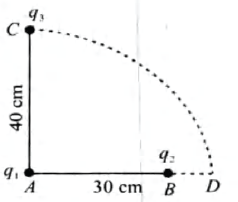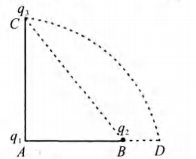# Q. Two charges $q_1$ and $q_2$ are placed 30 cm apart, as shown in the figure. A third charge $q_3$ is moved along the arc of a circle of radius 40cm from C to D. The change in the potential energy of the system is $\frac{q_3}{ 4 \pi \varepsilon_0}$ k. where k isAIPMTAIPMT 2005Electrostatic Potential And Capacitance

Solution:

## The potential energy when q3 is at point C $U_1 = \frac{1}{ 4 \pi \varepsilon_0} \bigg [ \frac{ q_1 q_3}{ 0.40} + \frac{ q_2 q_3}{ \sqrt{ (0 . 40)^2 + (0 . 30)^2 }} \bigg ]$ The potential energy when $q_3$ is at point D $U_2 = \frac{1}{ 4 \pi \varepsilon_0} \bigg [ \frac{ q_1 q_3}{ 0.40} + \frac{q_2 q_3 }{ 0.10} \bigg ]$ Thus change in potential energy is $\triangle U = U_2 - U_1$ $\Rightarrow \frac{q_3}{ 4 \pi \varepsilon_0} = k$ = $\frac{1}{ 4 \pi \varepsilon_0} \bigg [ \frac{ q_1 q_3}{ 0.40} + \frac{ q_2 q_3 }{ 0.10} - \frac{ q_1 q_3}{ 0 . 40} - \frac{ q_2 q_3 }{ 0 . 50 } \bigg ]$ $\Rightarrow k = \frac{ 5 q_2 - q_2 }{ 0 . 50 } = \frac{ 4q_2}{ 0 . 50} = 8 q_2$You must select option to get answer and solution

KCET 2004

KCET 2004

KCET 2005

KCET 2005

KCET 2005

KCET 2005

KCET 2006

KCET 2006

KCET 2009

## 1. The increase in pressure required to decrease the 200 L volume of a liquid by 0.004% (in kPa) is (Bulk modulus of the liquid ==2100 MPa)

Maharashtra CET 2006 Mechanical Properties Of Solids

## 2. To draw maximum current from a combination of cells, how should the cells be grouped?

AFMC 2006 Current Electricity

AFMC 2010 Atoms

NEET 2019 Waves

## 5. The period of revolution of planet A around the sun is 8 times that of B. The distance of A from the sun is how many times greater than that of B from the sun?

AIPMT 1997 Gravitation

## 6. The temperature of 100 g of water is to be raised from 24°C to 90°C by adding steam to it. The mass of the steam required for this purpose is

JIPMER 2015 Thermal Properties Of Matter

## 7. Two discs of same moment of inertia rotating about their regular axis passing through centre and perpendicular to the plane of disc with angular velocities $\omega_1$ and $\omega_2$ . They are brought into contact face to face coinciding the axis of rotation. The expression for loss of energy during this process is:-

NEET 2017 System Of Particles And Rotational Motion

## 8. The hot wire ammeter measures

Rajasthan PMT 2004 Alternating Current

## 9. A body of mass 4 kg is accelerated upon by a constant force, travels a distance of 5 m in the first second and a distance of 2 m in the third second. The force acting on the body is

KCET 2008 Laws Of Motion

## 10. The diameter of a flywheel is increased by 1%. Increase in its moment of inertia about the central axis is

JIPMER 2015 System Of Particles And Rotational Motion